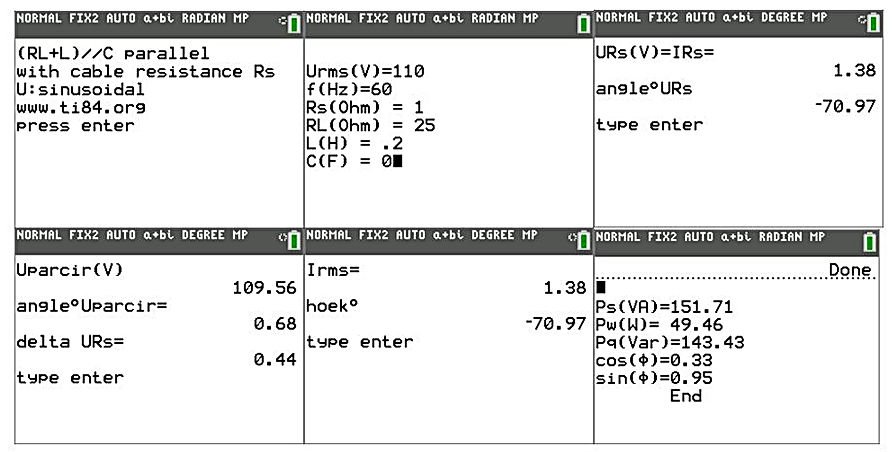naver-site-verification: naver47238a1b6bfbb19a2fd4b619734fa9a6.html
top of page

# Analysis of an AC parallel circuit using the Ti-84

## Two Programs, including a program which optimizes the power factor

The analyzed circuit is a very common electric circuit in electric engineering. See the diagrams below. In which RL and L is the representative electric load of electric equipment. In practice, many consumer equipments have an inductive character. The parameter Rs is the resistance of the cable and C1 is the capacitor which improves the cosφ. The source voltage is 110V_rms with a frequency of 60 Hz. This diagram can also be used for the one phase representation of a 3 phases symmetric load (all powers have to be multiplied with a factor3). Both schemes are analyzed with the program RLCPCRSE.8XP.

The left scheme is an inductive load (25 Ohm and 0.2H without Capacitor (0 F). In the right scheme, the inductive blind load is (over) compensated by using a capacitor of 40 uF. An extra program is added which automatically calculates the capacitor value to obtain a cosφ of 1.

See the results below.The original inductive behaviour of the circuit  without capacitor and with inductance of 0.2 H and resistance of 25 Ohm results in a cosφ=0.33, sinφ=0.95 (see results above).

A capacitor of 40 uF parallel to the inductive circuit changes cosφ to 0.79 and sinφ to 0.62.

Also, the source current decreases by more than a factor of 2 (1.38A to 0.55 A), the Watt power keeps almost the same (49.46 to 47.87 W) (see results below). This is a good example of how a capacitor influences the power factor.Notice that all parameters are free to choose, so a wide range of problems can be analyzed.

A program that calculates the capacitor value to achieve a cosφ=1

The program IMPROPF.8xp is demonstrated below. First, the original inductive circuit without a capacitor is analyzed. After that, the optimal capacitor value is calculated and  the optimal circuit is analyzed again## Calculation results original  system

The results show a cosφ of 0.57 which is not very high. The current is 1.2 A. The reactive power is 108.75 Var and the real power equals 75W. The voltage loss across Rs=2.4 V.## The calculation results of an optimized system with cosφ= 1

After analyzing the original inductive circuit, the program calculates the optimal capacitor value to improve the cosφ to 1. The optimized system shows a cosφ =1. The calculated capacitor equals 24.44uF. The source current decreases to 0.66A. The voltage loss across Rs decreases to 1.33V. The total reactive power =0Var.